# Properties of Light

#### Property of light

- The light from an object travels in a straight line.

There are two way to change the direction of light.

- Reflection

- Refraction

#### Reflection of light

When a light ray from a light source reaches on the surface of an object, the ray bounces off on it.

Then the ray enters our eyes. This is the reason why we can see an object. Especially, the ray bounces of in a regular way on the polished surface such as a mirror.

This bouncing phenomenon of light is called reflection of light .

- The ray that falls on the reflecting surface is called the incident ray .

- The ray that leaves the reflecting surface is called the reflected ray .

- The point where the ray strikes the reflecting surface is called the point of incidence .

- The line drawn at right angles to the reflecting surface at the point of incidence is called the normal .

- The angle between the incident ray and the normal is called the angle of incidence .

- The angle between the reflected ray and the normal is called the angle of reflection .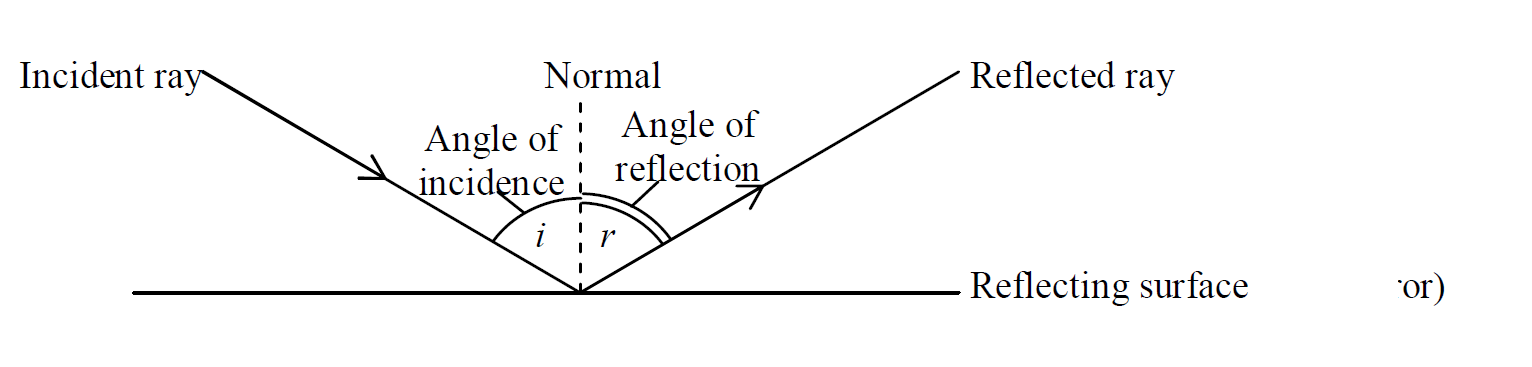#### Laws of Reflection

1. The angle of incidence is equal to the angle of reflection

i = r

2. The incident ray, the reflected ray and the normal all lie in the same plane.

#### Virtual image (in the plane mirror)

The image which cannot be formed on a screen is called the virtual image. You can see your virtual image in a mirror. In a plane mirror, the properties of the virtual image are shown below.

- The virtual image in a mirror is the same size as the object.

- The virtual image in a mirror appears at the same distance behind the mirror as the object is in front.

- The virtual image in a mirror is laterally inverted. (Right and left are opposite.)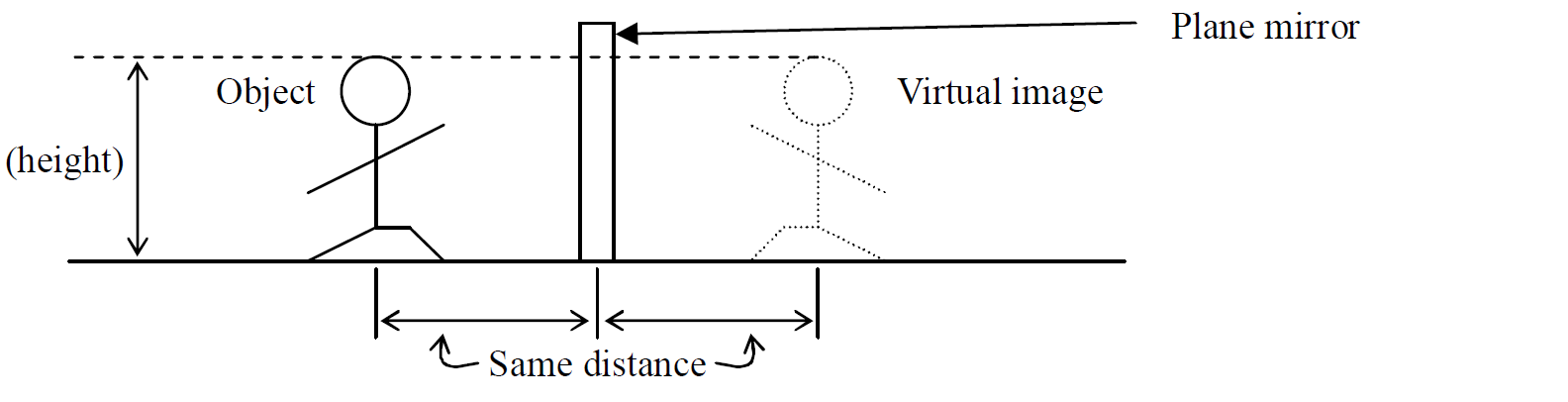Note that: The image which appears on a screen is called the real image. e.g. pine hole camera, movie

#### Laws of Refraction

The ratio of the sine of the angle of incidence to the sine of the angle of refraction is a constant.

Formula for refractive index

$\frac{\mathrm{Sin i}}{Sin r}=constant \left(n\right)$

The constant number ‘n’ is called the refractive index

This law is called Snell’s law

The incident ray, the reflected ray and the normal are in the same plane.

#### Example 1

Find the refractive index in the diagram below.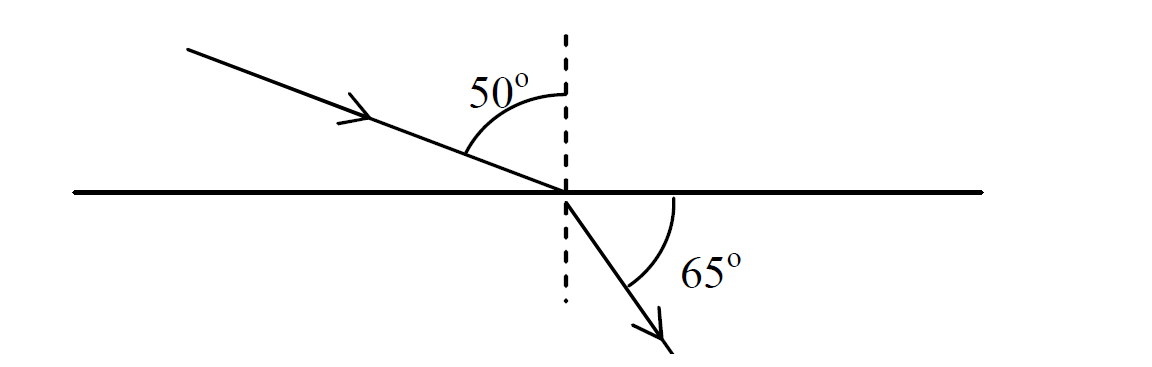Solution

$n=\frac{\mathrm{Sin i}}{Sin r}$

Given that

i = 50 o

r = 90-65 = 25 o

n = ?

$n=\frac{\mathrm{Sin 50}}{Sin 25}$

$n=\frac{\mathrm{0.77}}{0.42}$

Answer: $n=1.83$

#### Example 2

A ray of light travels from air into water at an angle of incidence 60 o. Find the angle of refraction if the refractive index of water is 1.33.

$n=\frac{\mathrm{Sin i}}{Sin r}$

Given that

i = 60o

r = ?

n = 1.33

$1.33=\frac{\mathrm{Sin 60}}{Sin r}$

$\mathrm{1.33 Sin r}=\mathrm{Sin 60}$

$\frac{\mathrm{1.33 Sin r}}{1.33}=\frac{\mathrm{Sin 60}}{1.33}$

$\mathrm{Sin r}=0.65$

${\mathrm{Sin r}}^{-1}=0.65$

$r={\mathrm{41}}^{o}$

#### Critical angle

Critical angle is the particular angle of incidence of a ray

hitting a less dense medium which results in it being refracted at 90°to the normal.

#### Thin converging lens

A lens that is thicker at the centre than at the edges is called a converging (convex) lens .

- The centre of lens is called the optical centre (C).

- The line passing through C and perpendicular to the plane of the lens is called the principal axis .

- The point where rays parallel to the principal axis converge is called the principal focus (F).

- The distance from the optical centre (C) to the principal focus (F) is called the focal length (f).

#### Rules to draw the ray diagram through lens

(1) Rays parallel to principal axis are refracted through F.

(2) Rays passing through F are refracted parallel to the principal axis

(3) Rays passing through C continue in a straight.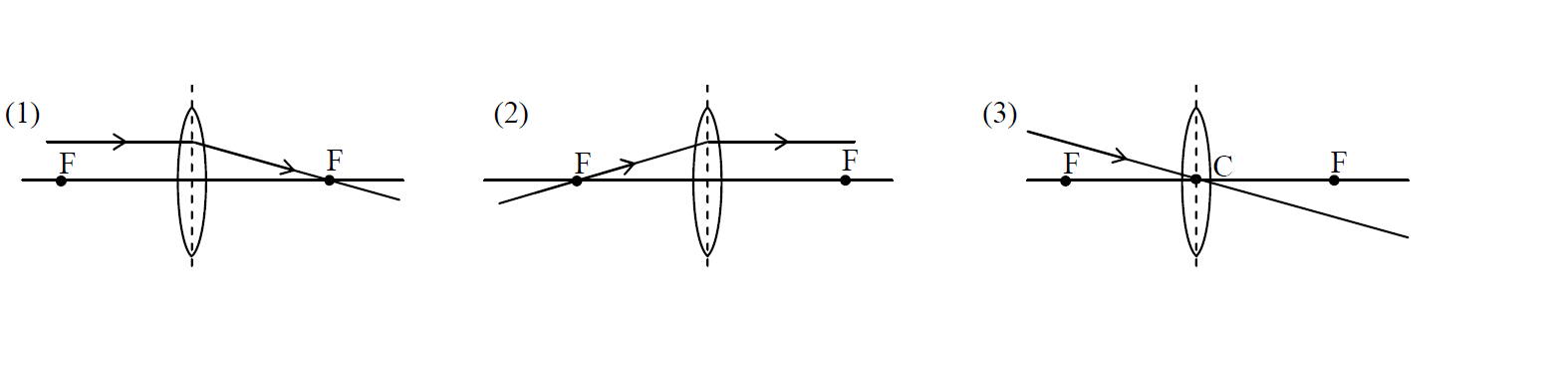#### Various image formed by convex lens

Object is between F and C.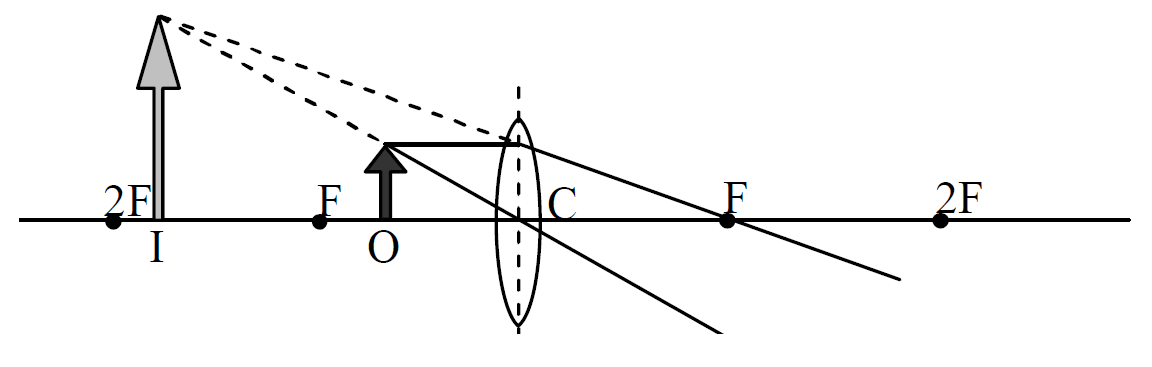- The image is between F and 2F.

- The image is virtual.

- The image is upright.

- The image is magnified.

Object is between 2F and F.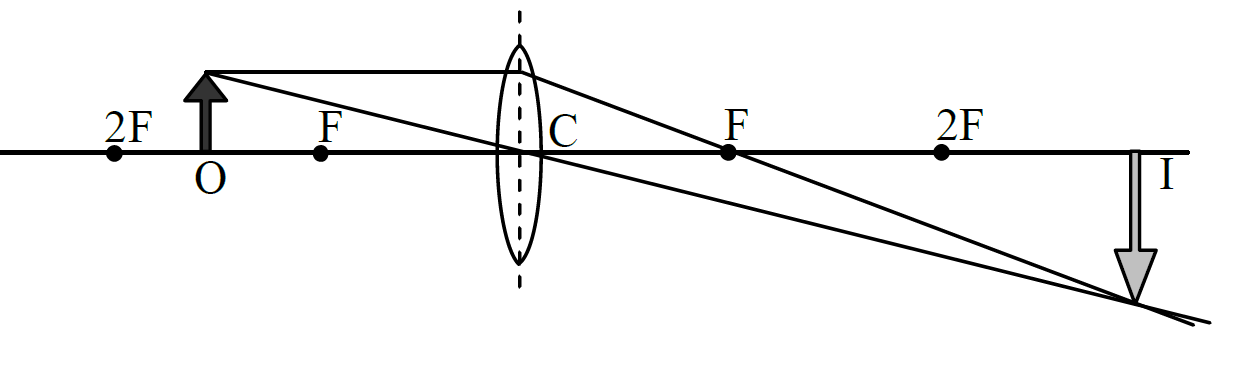- The image is beyond 2F.

- The image is real.

- The image is inverted.

- The image is magnified.

Object is beyond 2F.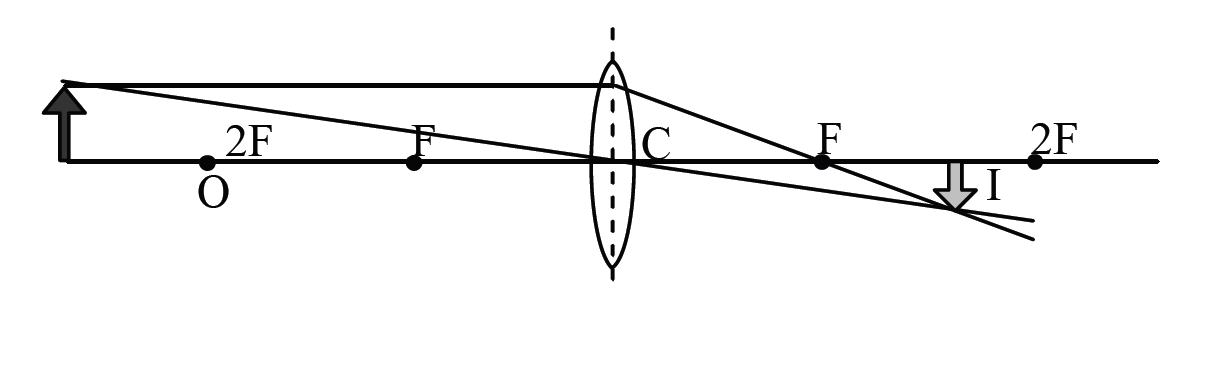- The image is between F and 2F.

- The image is real.

- The image is inverted

- The image is diminished.

#### Electromagnetic spectrum

Visible light consists of some colours, red, orange, yellow, green, blue, indigo and violet. When you see the rainbow, you can experience a light band. This band of coloured light is called the spectrum. The colours are in order of their wavelength.

And visible light is one of electromagnetic waves. Electromagnetic waves are named in the order of the ranging of wavelength. They are Radio waves, Microwaves, Infra-red rays (IR), Visible rays, Ultra-violet rays (UV), X-rays and γ(gamma)-rays. This band of electromagnetic waves is called the electromagnetic spectrum.

#### Properties of electromagnetic waves

Electromagnetic waves have some common properties shown below.

- They transfer energy from one place to another.

- They are all transverse waves.

- They can all travel through a vacuum.

- They all travel at 3×108m/s (300,000,000 m/s) in a vacuum.

- The equation (v=fλ) applies to all of them.

- γ-ray are the most energetic and the most penetrating rays. They are dangerous but also useful for the medical treatment such as radiotherapy.

- X-rays have a considerable penetrating power through matter and they also affect photographic films. These two properties make them suitable for use in seeing through objects, e.g. X-ray photographs for bones and metallic structure.

- Ultraviolet rays are sun emits a lot of ultraviolet rays. However, much of them is absorbed by a layer of ozone in the upper atmosphere of the earth. If much of them reaches our bodies, it can cause damages such as heavy sunburn, eye damage and skin cancer.

Infra-red rays It transfers heat energy but it is invisible. It can be detected by the infra-red photograph (which is used as Night photography) and the thermopile.

Microwaves are kinds of radio waves. They are used in radar systems, mirowave oven and the communication with satellites.